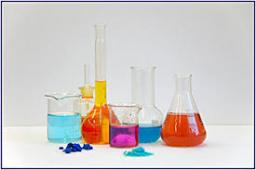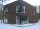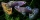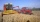# Alcohol mixture

From 55% and 80% alcohol we have to produce 0.2 kg of 60% alcohol. How many of them do we use in solution?

Result

a =  0.16
b =  0.04

#### Solution:

0.55a +0.80b = 0.60*0.20
a+b=0.2

0.55a+0.8b = 0.12
a+b = 0.2

a = 425 = 0.16
b = 125 = 0.04

Calculated by our linear equations calculator.Our examples were largely sent or created by pupils and students themselves. Therefore, we would be pleased if you could send us any errors you found, spelling mistakes, or rephasing the example. Thank you!

Leave us a comment of this math problem and its solution (i.e. if it is still somewhat unclear...):Be the first to comment!Tips to related online calculators
Do you have a system of equations and looking for calculator system of linear equations?

## Next similar math problems:

1. SolutionsHow much 60% solution and how much 35% solution is needed to create 100 l of 40% solution?
2. Three brothersThe three brothers have a total of 42 years. Jan is five years younger than Peter and Peter is 2 years younger than Michael. How many years has each of them?
3. ResearchersResearchers ask 200 families whether or not they were the homeowner and how many cars they had. Their response was homeowner: 14 no car or one car, two or more cars 86, not homeowner: 38 no car or one car, two or more cars 62. What percent of the families
4. Guppies for salePaul had a bowl of guppies for sale. Four customers were milling around the store. 1. Rod told paul - I'll take half the guppies in the bowl, plus had a guppy. 2. Heather said - I'll take half of what you have, plus half a guppy. The third customer, Na
5. PotatoesFor three days the store sold 1400 kg of potatoes. The first day they sold 100 kilograms of potatoes less than the second day, the third-day three-fifths of what they sold the first day. How many kgs of potatoes sold every day?
6. The farmThe farm harvested 390 tons of grain. Wheat was 15% more than barley, and rye was 126 tons less than wheat and barley combined. How many tons of wheat, how many tons of barley, and how much rye did the team get?
7. TheatroTheatrical performance was attended by 480 spectators. Women were in the audience 40 more than men and children 60 less than half of adult spectators. How many men, women and children attended a theater performance?
8. The ballThe ball was discounted by 10 percent and then again by 30 percent. How many percent of the original price is now?
9. The Chemistry testThe Chemistry test contained 8 questions, each with 3 points. Peter scored 21 points. How many percent did Peter write a test?
10. SummerjobThe temporary workers planted new trees. Of the total number of 500 seedlings, they managed to plant 426. How many percents did they meet the daily planting limit?
11. The dormitoryThe dormitory accommodates 150 pupils in 42 rooms, some of which are triple and some are quadruple. Determine how many rooms are triple and how many quadruples.
12. Three workshopsThere are 2743 people working in three workshops. In the second workshop works 140 people more than in the first and in third works 4.2 times more than the second one. How many people work in each workshop?
13. Apple varieteThis year, my uncle harvested 350 kg of apples, including 105 kilograms of Ontario, and the rest is Jonathan. Determine what percentage of the Jonathan apples.
14. Percentage increaseIncrease number 400 by 3.5%
15. Elimination methodSolve system of linear equations by elimination method: 5/2x + 3/5y= 4/15 1/2x + 2/5y= 2/15
16. Two equationsSolve equations (use adding and subtracting of linear equations): -4x+11y=5 6x-11y=-5
17. Linsys2Solve two equations with two unknowns: 400x+120y=147.2 350x+200y=144